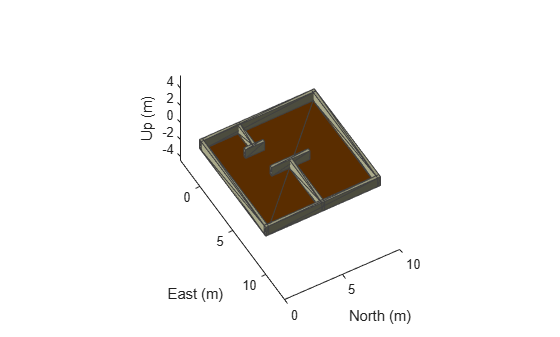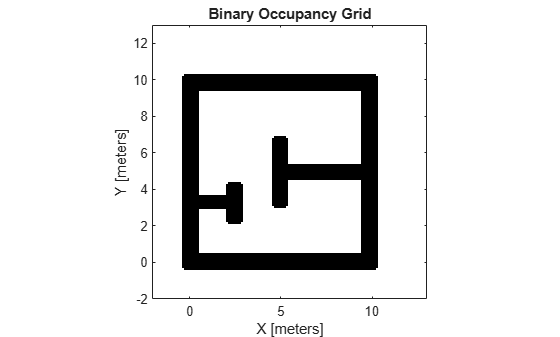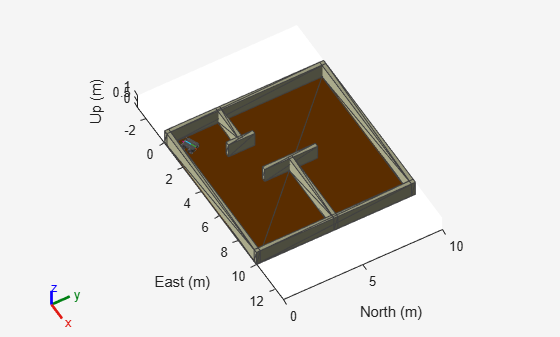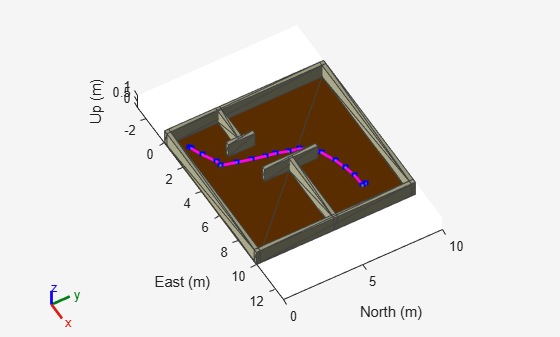# Perform Path Planning Simulation with Mobile Robot

Create a scenario to simulate a mobile robot navigating a room. The example demonstrates how to create a scenario, model a robot platform from a rigid body tree object, obtain a binary occupancy grid map from the scenario, and plan a path for the mobile robot to follow using the `mobileRobotPRM` path planning algorithm.

### Create Scenario with Ground Plane and Static Meshes

A `robotScenario` object consists of a set of static obstacles and movable objects called platforms. Use `robotPlatform` object to model the mobile robot within the scenario. This example builds a scenario consisting of a ground plane and box meshes to create a room.

`scenario = robotScenario(UpdateRate=5);`

Add a plane mesh as ground plane in the scenario.

```floorColor = [0.5882 0.2941 0]; addMesh(scenario,"Plane",Position=[5 5 0],Size=[10 10],Color=floorColor);```

The walls of the room are modeled as box meshes. The static meshes are added with the `IsBinaryOccupied` value set to `true`, so these obstacles are incorporated into the binary occupancy map used for path planning.

```wallHeight = 1; wallWidth = 0.25; wallLength = 10; wallColor = [1 1 0.8157]; % Add outer walls. addMesh(scenario,"Box",Position=[wallWidth/2, wallLength/2, wallHeight/2],... Size=[wallWidth, wallLength, wallHeight],Color=wallColor,IsBinaryOccupied=true); addMesh(scenario,"Box",Position=[wallLength-wallWidth/2, wallLength/2, wallHeight/2],... Size=[wallWidth, wallLength, wallHeight],Color=wallColor,IsBinaryOccupied=true); addMesh(scenario,"Box",Position=[wallLength/2, wallLength-wallWidth/2, wallHeight/2],... Size=[wallLength, wallWidth, wallHeight],Color=wallColor,IsBinaryOccupied=true); addMesh(scenario,"Box",Position=[wallLength/2, wallWidth/2, wallHeight/2],... Size=[wallLength, wallWidth, wallHeight],Color=wallColor,IsBinaryOccupied=true); % Add inner walls. addMesh(scenario,"Box",Position=[wallLength/8, wallLength/3, wallHeight/2],... Size=[wallLength/4, wallWidth, wallHeight],Color=wallColor,IsBinaryOccupied=true); addMesh(scenario,"Box",Position=[wallLength/4, wallLength/3, wallHeight/2],... Size=[wallWidth, wallLength/6, wallHeight],Color=wallColor,IsBinaryOccupied=true); addMesh(scenario,"Box",Position=[(wallLength-wallLength/4), wallLength/2, wallHeight/2],... Size=[wallLength/2, wallWidth, wallHeight],Color=wallColor,IsBinaryOccupied=true); addMesh(scenario,"Box",Position=[wallLength/2, wallLength/2, wallHeight/2],... Size=[wallWidth, wallLength/3, wallHeight],Color=wallColor,IsBinaryOccupied=true);```

Visualize the scenario.

```show3D(scenario); lightangle(-45,30); view(60,50);```### Obtain Binary Occupancy Map from Scenario

Obtain an occupancy map as a binaryOccupancyMap object from the scenario for path planning. Inflate the occupied spaces on the map by 0.3m.

```map = binaryOccupancyMap(scenario,GridOriginInLocal=[-2 -2],... MapSize=[15 15],... MapHeightLimits=[0 3]); inflate(map,0.3);```

Visualize the 2-D occupancy map.

`show(map)`### Path Planning

Use the `mobileRobotPRM` path planner to find an obstacle-free path between the start and goal positions on the obtained map.

Specify the start and goal positions of the mobile robot.

```startPosition = [1 1]; goalPosition = [8 8];```

Set the `rng` seed for repeatability.

`rng(100)`

Create a `mobileRobotPRM` object with the binary occupancy map and specify the maximum number of nodes. Specify the maximum distance between the two connected nodes.

```numnodes = 2000; planner = mobileRobotPRM(map,numnodes); planner.ConnectionDistance = 1;```

Find a path between the start and goal positions.

`waypoints = findpath(planner,startPosition,goalPosition);`

### Trajectory Generation

Generate trajectory for the mobile robot to follow with the waypoints from the planned path using the `waypointTrajectory` System object.

```% Robot height from base. robotheight = 0.12; % Number of waypoints. numWaypoints = size(waypoints,1); % Robot arrival time at first waypoint. firstInTime = 0; % Robot arrival time at last waypoint. lastInTime = firstInTime + (numWaypoints-1); % Generate waypoint trajectory with waypoints from planned path. traj = waypointTrajectory(SampleRate=10,... TimeOfArrival=firstInTime:lastInTime, ... Waypoints=[waypoints, robotheight*ones(numWaypoints,1)], ... ReferenceFrame="ENU");```

### Add Robot Platform to Scenario

Load the Clearpath Husky mobile robot from the robot library as a `rigidBodyTree` object.

`huskyRobot = loadrobot("clearpathHusky");`

Create a `robotPlatform` object with the robot model specified as a `rigidBodyTree` object and its trajectory specified as a `waypointTrajectory` System object.

```platform = robotPlatform("husky",scenario, RigidBodyTree=huskyRobot,... BaseTrajectory=traj);```

Visualize the scenario with the robot.

```[ax,plotFrames] = show3D(scenario); lightangle(-45,30) view(60,50)```### Simulate Mobile Robot

Visualize the planned path.

```hold(ax,"on") plot(ax,waypoints(:,1),waypoints(:,2),"-ms",... LineWidth=2,... MarkerSize=4,... MarkerEdgeColor="b",... MarkerFaceColor=[0.5 0.5 0.5]); hold(ax,"off")```

Set up the simulation. Since all the poses of the robot are known in advance, simply step through the simulation and update the visualization at each step.

```setup(scenario) % Control simulation rate at 20 Hz. r = rateControl(20); % Status of robot in simulation. robotStartMoving = false; while advance(scenario) show3D(scenario,Parent=ax,FastUpdate=true); waitfor(r); currentPose = read(platform); if ~any(isnan(currentPose)) % implies that robot is in the scene and performing simulation. robotStartMoving = true; end if any(isnan(currentPose)) && robotStartMoving % break, once robot reaches goal position. break; end end```To re-run the simulation and visualize the results again, reset the simulation in the scenario.

`restart(scenario)`